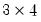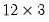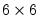Command Reference : Matrix Language Reference

 @unvec
Unstack vector into a matrix.
Syntax: @unvec(v, n)
v: vector
n: integer
Return: matrix
Creates an n-row matrix filled with the unstacked elements of the vector v. EViews will report a size mismatch if the number of elements of v is not evenly divisible by n.
Note that @unvec is the inverse of the @vec function.
Examples
vector v = @mrnd(12)
matrix m1 = @unvec(v1, 3)
creates a 12 element vector of uniform random numbers V1 and unstacks it into amatrix M1.
matrix m2 = @mnrnd(12, 3)
matrix m3 = @unvec(@vec(m2), 6)
generates amatrix of random normals M2, then reshapes the matrix into amatrix M3.
Cross-references